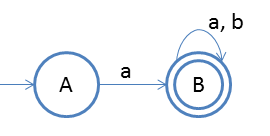## Conversion of Finite Automata to Right Linear Regular Grammar

Converting finite automata to right lienar grammar is very simple
See below steps and example followed by it, we will understand the process.

1. Repeat the process for every state
2. Begin the process from start state
3. Write the production as the output followed by the state on which the transition is going
4. And at the last add ε because that's is required to end the derivation
Note:We have added ε because either you could continue the derivation or would like to stop it. So to stop the derivation we have written εSo we will applt the above procedure
Pick start state and output is on symbol 'a' we are going on state B
So we will write as :
```
A -> aB
```
And then we will pick state B and then we will go for each output.
so we will get the below production.
```
B -> aB/bB/ε
```

So final we got right linear grammar as:
```
A -> aB
B -> aB/bB/ε
```